• 求出对角线之和！ 只需要修改定义的define z的值就好！ 接下来，进入正题！ 题目描述： 求一个3×3矩阵对角线元素之和。 输入 矩阵 输出 主对角线 副对角线 元素和 样例输入 1 2 3 1 1 1 3 2 1 ...
这个我做了改进，可以实现NXN的矩阵。求出对角线之和！ 只需要修改定义的define z的值就好！ 接下来，进入正题！
题目描述：
求一个3×3矩阵对角线元素之和。

输入
矩阵

输出
主对角线 副对角线 元素和

样例输入
1 2 3
1 1 1
3 2 1

样例输出
3 7

来源/分类
C语言

题目截图：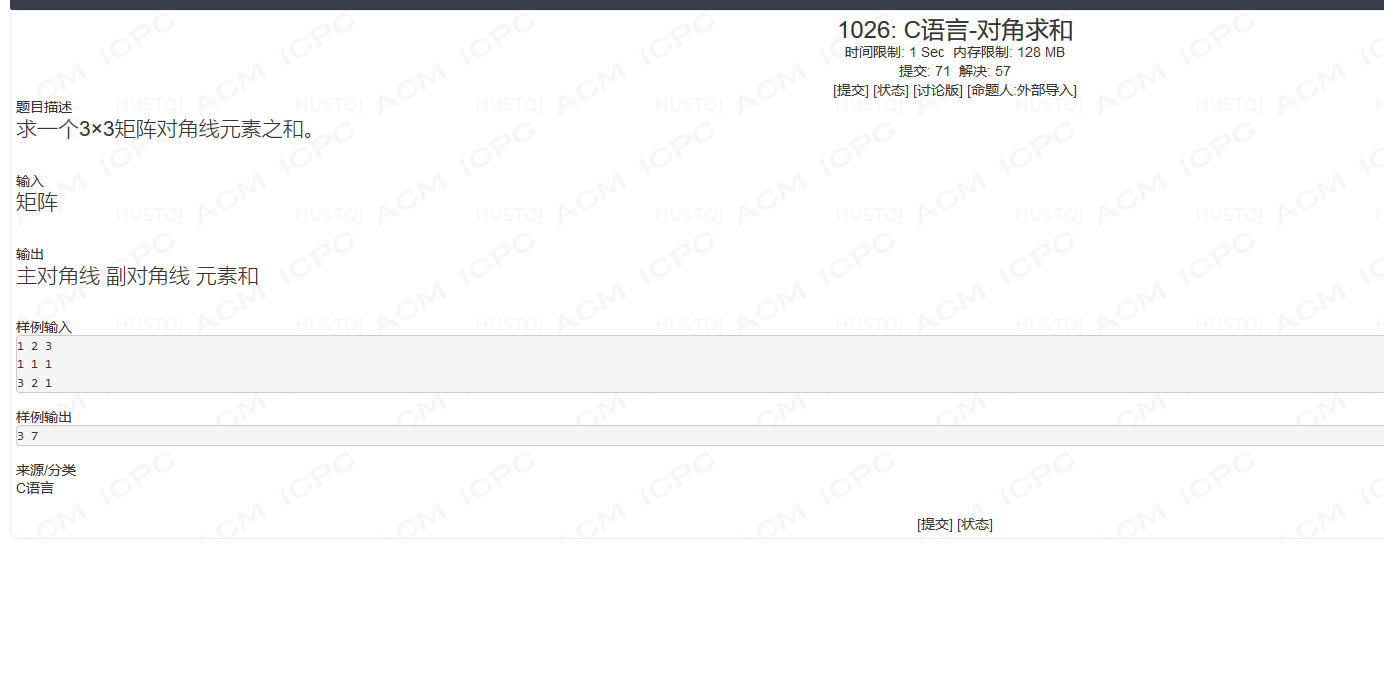思路：
直接修改#define z
修改后面的数值，就可以改变矩阵的大小啦！
本例子给出的是矩阵为4！

先来一个输入的程序：
for(i=0;i<z;i++)
{
for(j=0;j<z;j++)
{
cin>>a[i][j];
}
}
接下来进行对角线求和！
两个下标相等的是：
if(i==j)
{s+=a[i][j];}
然后是斜对角线的相加！
if((i+j)==z-1)//下标减去1！就是斜对角线！
{w+=a[i][j];}
然后就OK啦！

代码：
#include<iostream>
using namespace std;
#define z 4
int main()
{
int a[z][z]={0};int i,j;int s=0;int w=0;
for(i=0;i<z;i++)
{
for(j=0;j<z;j++)
{
cin>>a[i][j];
}
}
for(i=0;i<z;i++)
{
for(j=0;j<z;j++)
{
if(i==j)
{s+=a[i][j];}
if((i+j)==z-1)
{w+=a[i][j];}

}
//cout<<endl;
}
cout<<s<<" "<<w;
}

代码截图：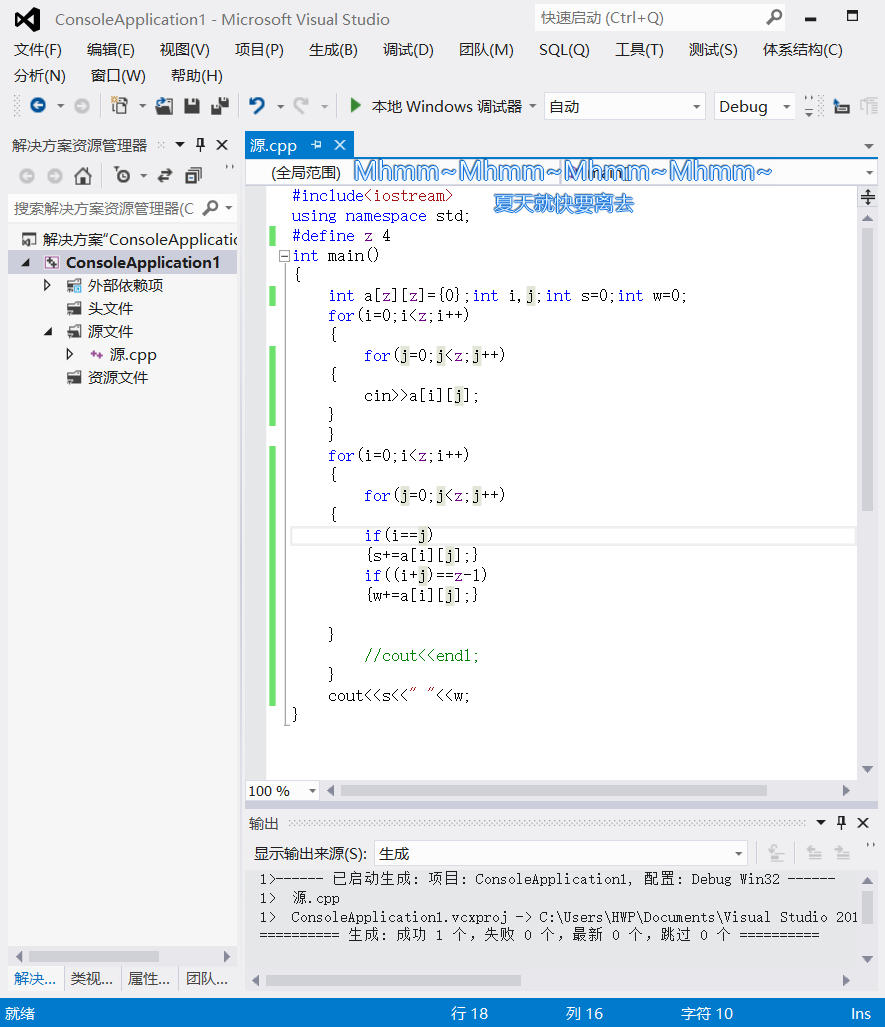运行结果：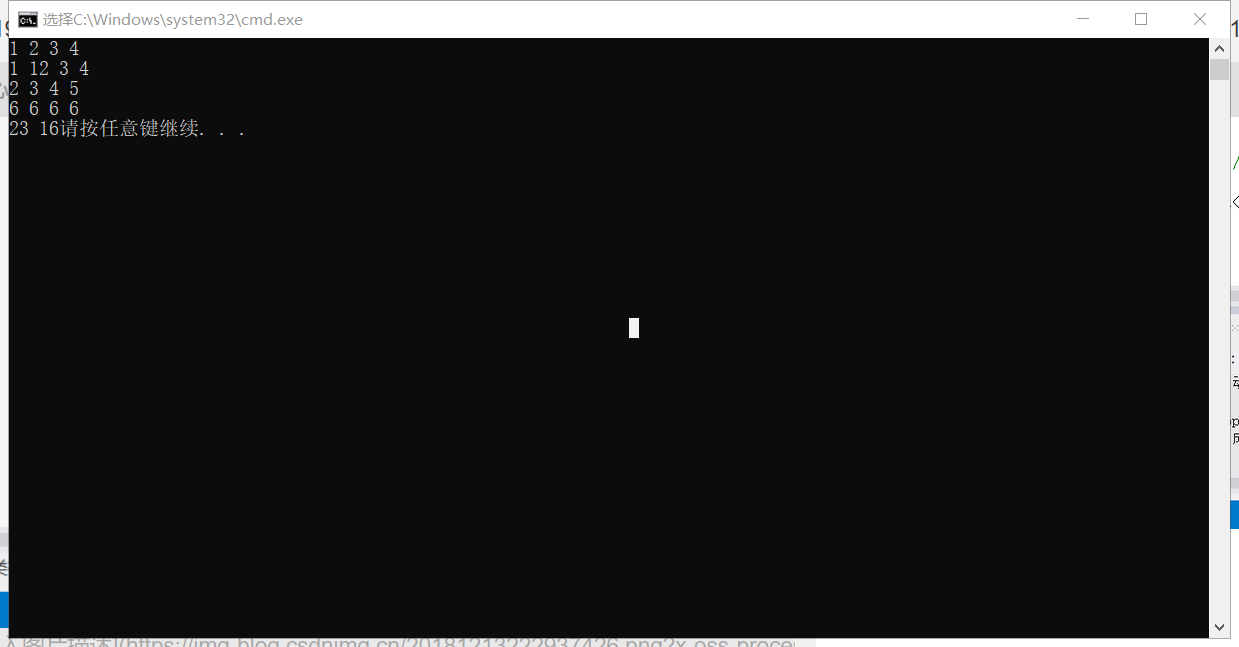OJ结果：展开全文• - [矩阵对角化](#矩阵对角化) - [SVD分解](#svd分解) - [参考链接](#参考链接) 矩阵对角化 矩阵的相似 设 A\boldsymbol{A}A、 B\boldsymbol{B}B 为两个nnn阶矩阵，若存在可逆矩阵 P\boldsymbol{P}P，使得...


文章目录
矩阵对角化SVD分解参考链接

矩阵对角化
矩阵的相似

设

A

\boldsymbol{A}

、

B

\boldsymbol{B}

为两个

n

n

阶矩阵，若存在可逆矩阵

P

\boldsymbol{P}

，使得

P

−

1

A

P

=

B

\boldsymbol{P}^{^{-1}}\boldsymbol{A}\boldsymbol{P}=\boldsymbol{B}

则称

A

\boldsymbol{A}

与

B

\boldsymbol{B}

相似。特别的，如果

B

\boldsymbol{B}

为对角形矩阵，则称

A

\boldsymbol{A}

可(相似)对角化

n

n

阶矩阵

A

A

可对角化的充要条件：

A

\boldsymbol{A}

有

n

n

个线性无关的特征向量

X

1

,

X

2

,

.

.

.

X

n

\boldsymbol{X_{1}},\boldsymbol{X_{2}},...\boldsymbol{X_{n}}

。具体的证明我们不在此展开，只做几点说明：
设

n

n

阶矩阵

A

\boldsymbol{A}

有

m

m

个不同特征值（方程

∣

λ

E

−

A

∣

=

0

\left | \lambda \boldsymbol{E} -\boldsymbol{A}\right |=0

有

m

m

个不同实根），则不同特征值对应的特征向量线性无关存在

A

\boldsymbol{A}

属于某个特征值的线性无关特征向量当

P

−

1

A

P

=

d

i

a

g

(

λ

1

，

λ

2

，

.

.

.

λ

n

)

\boldsymbol{P}^{^{-1}}\boldsymbol{A}\boldsymbol{P}=diag(\lambda _{1}，\lambda _{2}，...\lambda _{n})

时，

d

i

a

g

(

λ

1

，

λ

2

，

.

.

.

λ

n

)

diag(\lambda _{1}，\lambda _{2}，...\lambda _{n})

的

n

n

个主对角元是

A

\boldsymbol{A}

的

n

n

个特征值（含重根）；

A

\boldsymbol{A}

分别属于

λ

1

，

λ

2

，

.

.

.

λ

n

\lambda _{1}，\lambda _{2}，...\lambda _{n}

的线性无关特征向量

X

1

,

X

2

,

.

.

.

X

n

\boldsymbol{X_{1}},\boldsymbol{X_{2}},...\boldsymbol{X_{n}}

构成了可逆矩阵

P

\boldsymbol{P}

的列向量
实对称矩阵的对角化
考虑实对称矩阵的对角化，有如下结论：

n

n

阶实对称矩阵

A

\boldsymbol{A}

的特征值都是实数（证明思路：对表达式

A

X

=

λ

X

\mathbf{AX=\lambda X}

左乘复特征向量的共轭转置

X

ˉ

T

\mathbf{\bar{X}^{T}}

，推出

λ

\lambda

为实数）实对称矩阵

A

\boldsymbol{A}

不同特征值对应的特征向量正交（证明思路：列出

A

\boldsymbol{A}

两组不同的特征方程，分别左乘另一个复特征向量的共轭转置，两端取转置后方程相减）属于

A

\boldsymbol{A}

的同一个特征值的一组线性无关特征向量不一定正交，但可用施密特正交方法将其正交化一定存在正交矩阵

Q

\mathbf{Q}

（满足

Q

−

1

=

Q

T

\mathbf{Q}^{-1}=\mathbf{Q}^{T}

）,使得

Q

−

1

A

Q

\boldsymbol{Q}^{^{-1}}\boldsymbol{A}\boldsymbol{Q}

为对角形矩阵

n

n

阶实对称矩阵

A

\boldsymbol{A}

存在

n

n

个正交的单位特征向量
SVD分解
特征值分解只能用于可逆方阵，奇异值分解（SVD）适用于任意

m

×

n

\mathit{m}\times\mathit{n}

矩阵，定义如下：

若

A

\boldsymbol{A}

是一个

m

×

n

\mathit{m}\times\mathit{n}

矩阵，则存在一个分解，使得

A

=

U

Σ

V

∗

\boldsymbol{A}=\boldsymbol{U}\Sigma\boldsymbol{V}^{*}

其中

U

\boldsymbol{U}

是

m

×

m

m\times m

酉矩阵（满足

U

−

1

=

U

∗

\boldsymbol{U^{-1}}=\boldsymbol{U}^{*}

）,

Σ

\Sigma

是

m

×

n

m\times n

非负实对称矩阵，

V

∗

\boldsymbol{V}^{*}

是

V

\boldsymbol{V}

的共轭转置，是

n

×

n

n\times n

酉矩阵.

求解

U

\boldsymbol{U}

,

Σ

\Sigma

,

V

\boldsymbol{V}

矩阵
给定一个

A

m

×

n

\boldsymbol{A_{m\times n}}

的奇异值分解，有：

A

∗

A

=

V

Σ

∗

U

∗

U

Σ

V

∗

=

V

(

Σ

∗

Σ

)

V

∗

\boldsymbol{A^{*}A}=\boldsymbol{V}\Sigma^{*}\boldsymbol{U}^{*}\boldsymbol{U}\Sigma\boldsymbol{V}^{*}=\boldsymbol{V}(\Sigma^{*}\Sigma)\boldsymbol{V}^{*}

A

A

∗

=

U

Σ

V

∗

V

Σ

∗

U

∗

=

U

(

Σ

Σ

∗

)

U

∗

\boldsymbol{AA^{*}}=\boldsymbol{U}\Sigma\boldsymbol{V}^{*}\boldsymbol{V}\Sigma^{*}\boldsymbol{U}^{*}=\boldsymbol{U}(\Sigma\Sigma^{*})\boldsymbol{U}^{*}

关系式右边描述了关系式左边的特征值分解，于是：

A

∗

A

\boldsymbol{A^{*}A}

的

n

n

个特征向量（右奇异向量）组成了

V

\boldsymbol{V}

的列向量

A

A

∗

\boldsymbol{AA^{*}}

的

m

m

个特征向量（左奇异值向量）组成了

U

\boldsymbol{U}

的列向量

Σ

\Sigma

的非零对角元（非零奇异值）是

A

∗

A

\boldsymbol{A^{*}A}

或

A

A

∗

\boldsymbol{AA^{*}}

的非零特征值（为啥相同？）的平方根，即

Σ

=

[

σ

1

0

0

0

0

σ

2

⋯

0

0

0

⋱

σ

r

⋮

⋮

⋮

⋮

]

m

×

n

\Sigma=\begin{bmatrix} \sigma_{1}& 0& 0& 0 \\ 0& \sigma_{2}& \cdots& 0 \\ 0& 0& \ddots& \sigma_{r} \\ \vdots& \vdots& \vdots& \vdots \end{bmatrix}_{m\times n}

，其中

r

=

r

a

n

k

(

A

)

r=rank(\boldsymbol{A})

（思考为什么？）且

m

>

r

\mathit{m}>\mathit{r}

，

σ

i

=

λ

i

(

i

=

1

,

2

⋯

r

)

\sigma _{i}=\sqrt{\lambda _{i}}(i=1,2\cdots r)

补充1：

A

A

T

\boldsymbol{AA^{T}}

性质
对称性：

(

A

T

A

)

T

=

A

A

T

(\boldsymbol{A^{T}A})^{T}=\boldsymbol{AA^{T}}

半正定性：对任意非零向量

x

n

×

1

x_{n\times1}

，有

x

T

(

A

T

A

)

x

=

(

A

x

)

T

(

A

x

)

⩾

0

\boldsymbol{x^{T}}(\boldsymbol{A^{T}A})\boldsymbol{x}=(\boldsymbol{Ax})^{T}(\boldsymbol{Ax})\geqslant 0

补充2：奇异值分解 Vs.特征值分解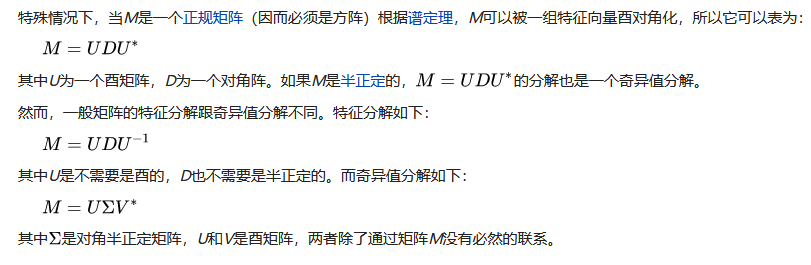补充3：正定和半正定矩阵的性质
正定矩阵的行列式恒为正；实对称矩阵

A

A

正定当且仅当

A

A

与单位矩阵合同；对于

n

n

阶实对称矩阵

A

A

，下列条件是等价的：正定矩阵=一切主子式均为正=一切顺序主子式均为正=特征值均为正；对于

n

n

阶实对称矩阵

A

A

，下列条件是等价的：半正定矩阵=所有的主子式非负（顺序主子式非负并不能推出矩阵是半正定的）
SVD分解的应用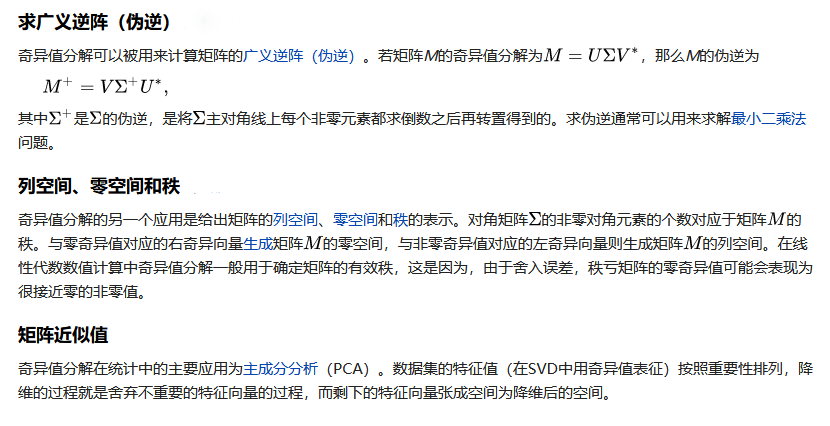参考链接
中文维基 奇异值分解中文维基 可对角化矩阵博客园 奇异值分解(SVD)原理与在降维中的应用知乎 矩阵对角化与奇异值分解Markdown语言教程 Markdown 插入链接 Markdown 编辑器语法指南 MarkDown 插入数学公式实验大集合 如何在Markdown中写公式
展开全文线性代数 SVD
• 对角矩阵：M是一个对角矩阵，当且仅当i!=l时，M(i,j)=0 三对角矩阵：M是一个三对角矩阵，当且仅当|i-j|>1时，M(i,j)=0 下三角矩阵：M是一个下三角矩阵，当且仅当i<j时，M(i,j)=0 上三角矩阵：M是一个上...
一、特殊矩阵（方阵）
方阵：是指行数与列数相同的矩阵一些常用的特殊方阵如下：
对角矩阵：M是一个对角矩阵，当且仅当i!=l时，M(i,j)=0三对角矩阵：M是一个三对角矩阵，当且仅当|i-j|>1时，M(i,j)=0下三角矩阵：M是一个下三角矩阵，当且仅当i<j时，M(i,j)=0上三角矩阵：M是一个上三角矩阵，当且仅当i>j时，M(i,j)=0对称矩阵：M是一个对称矩阵，当且仅当对于所有的j和j，M(i,j)=M(j,i)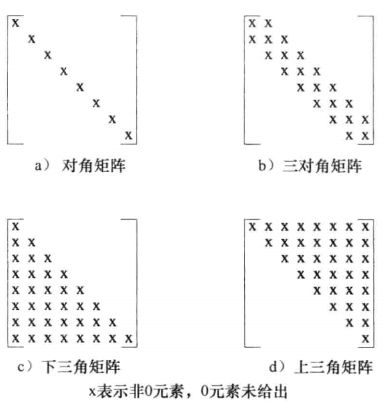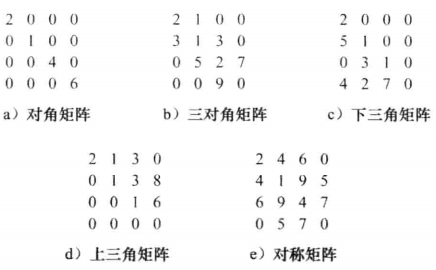二、特殊矩阵（方阵）在实际中的应用

应用①
佛罗里达州的6个城市Gainsville、Jacksonville、Miami、Orlando、Tallaha-ssee和Tampa按照这个顺序，依次从1~6编号。任意两个城市之间的距离用一个6*6的矩阵distance表示。矩阵的第i行和第i列代表第i个城市。distance(i,j)代表城市i和城市j之间的距离下图给出了相应的矩阵，因为对于所有的i和j有distance(i,j)=distance(j,i)，所以这是一个对称矩阵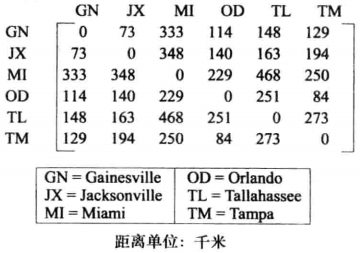应用②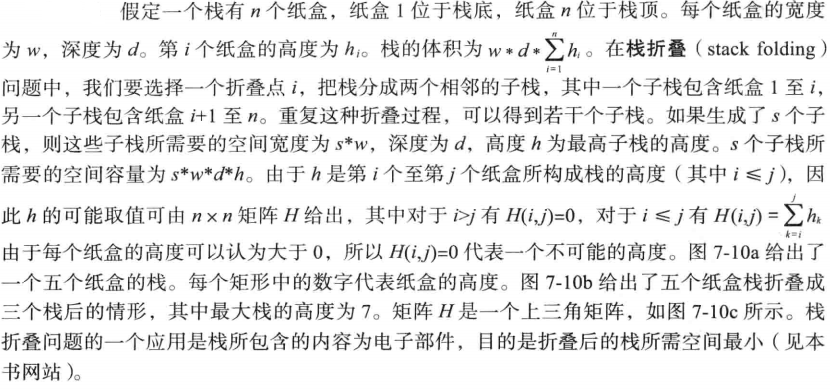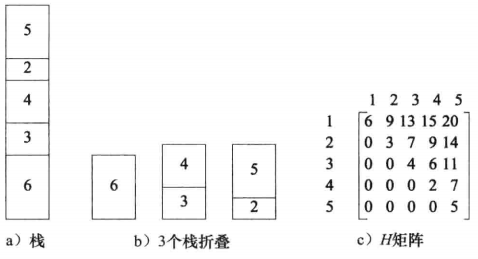三、对角矩阵
对角矩阵：M是一个对角矩阵，当且仅当i!=l时，M(i,j)=0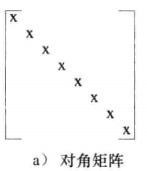编码实现：
一个rows*rows的对角矩阵D可以表示为一个二维数组element[rows][rows]，其中element[i-1][j-1]=D(i,i)。这种表示法需要rows*rows个类型为T的数据空间然后对角矩阵最多只有rows个非0元素，因此可以用一位数组element[rows]来表示，其中element[i-1]=D(i,i)，所有未在一维数组中出现的矩阵元素均为0
异常类定义
class illegalParameterValue
{
string message;
public:
illegalParameterValue(const char *theMessage ="Illegal Parameter Value"):message(theMessage) {}
const char *what() {
return message.c_str();
}
};

class matrixIndexOutOfBounds
{
public:
matrixIndexOutOfBounds(string theMessage = "Matrix index out of bounds") :message(theMessage){}
const char *what() {
return message.c_str();
}
private:
string message;
};
类定义
template<typename T>
class diagonalMatrix
{
public:
diagonalMatrix(int theN = 10);
~diagonalMatrix();
void set(int i, int j, T value);
T get(int i, int j)const;
void output(ostream& out) const;
private:
T *element;  //存放矩阵的数组
int n;  //矩阵维度
};
类成员实现
构造函数时间复杂度：当T时内部数据类型时为O(1)，当T为用户定义类型时为O(rows)get、set的时间复杂度：Θ(1)
template<typename T>
diagonalMatrix<T>::diagonalMatrix(int theN = 10)
{
if (theN < 1) {
throw illegalParameterValue("Parameter mu be >=1");
}

element = new T[theN];
n = theN;
}

template<typename T>
diagonalMatrix<T>::~diagonalMatrix()
{
if (element) {
delete[] element;
element = nullptr;
}
}

template<typename T>
void diagonalMatrix<T>::set(int i, int j, T value)
{
if ((i<1) || (j<1) || (i>n) || (j>n)) {
throw matrixIndexOutOfBounds();
}

if (i == j) {
element[i] = value;
}
else {
if (value != 0) {
throw illegalParameterValue("nondiagonal elements must be zero");
}
}
}

template<typename T>
T diagonalMatrix<T>::get(int i, int j)const
{
if ((i<1) || (j<1) || (i>n) || (j>n)) {
throw matrixIndexOutOfBounds();
}

if (i == j)
return element[i];
else
return 0;
}

template<typename T>
void diagonalMatrix<T>::output(ostream& out) const
{
for (int i = 1; i <= n; ++i) {
for (int j = 1; j <= n; j++) {
if (i == j) {
out << element[i]<<" ";
continue;
}
out << "0 ";
}
out << endl;
}
}
演示案例
int main()
{
diagonalMatrix<int> *matrix = new diagonalMatrix<int>(3);

matrix->set(1,1,1);
matrix->set(2, 2, 2);
matrix->set(3, 3, 3);

cout << "matrix[1,1]="<< matrix->get(1, 1) <<endl;
cout << "matrix[2,2]=" << matrix->get(2, 2) << endl;
cout << "matrix[3,3]=" << matrix->get(3, 3) << endl;

matrix->output(cout);

return 0;
}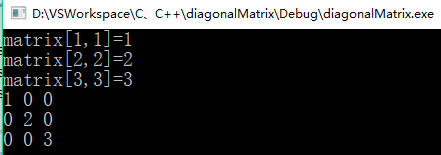四、三对角矩阵
三对角矩阵：M是一个三对角矩阵，当且仅当|i-j|>1时，M(i,j)=0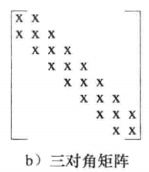非0元素排列在如下三条对角线上：
①主对角线：i=j②主对角线之下的对角线（称为低对角线）：i=j+1③主对角线之上的对角线（称为高对角线）：i=j-1

编码实现：
三条对角线上的元素总数为3*rows-2。可以用一个容量3*rows-2的一维数组element来描述三对角矩阵以下图这个三对角矩阵为例：
如果逐行映射：则element[0-9]={2,1,3,1,3,5,7,9,0}如果逐列映射：则element[0-9]={2,3,1,1,5,3,2,9,7,0}如果从低对角线开始逐条对角线映射：则element[0-9]={3,5,9,2,1,2,0,1,3,7}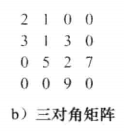每一种映射方式，get和set方法的代码都不同，下面我们假设为逐条对角线映射
异常类定义
class illegalParameterValue
{
string message;
public:
illegalParameterValue(const char *theMessage ="Illegal Parameter Value"):message(theMessage) {}
const char *what() {
return message.c_str();
}
};

class matrixIndexOutOfBounds
{
public:
matrixIndexOutOfBounds(string theMessage = "Matrix index out of bounds") :message(theMessage){}
const char *what() {
return message.c_str();
}
private:
string message;
};
类定义
template<typename T>
class tridiagonalMatrix
{
public:
tridiagonalMatrix(int theN = 10);
~tridiagonalMatrix();
T get(int i,int j)const;
void set(int i, int j, T value);
void output(ostream& out) const;
private:
T *element;  //存放矩阵的数组
int n; //矩阵维数
};
类成员实现
template<typename T>
tridiagonalMatrix<T>::tridiagonalMatrix(int theN = 10)
{
if (theN < 1) {
throw illegalParameterValue("Parameter mu be >=1");
}

element = new T[3*theN-2];
n = theN;
}

template<typename T>
tridiagonalMatrix<T>::~tridiagonalMatrix()
{
if (element) {
delete[] element;
element = nullptr;
}
}

template<typename T>
T tridiagonalMatrix<T>::get(int i, int j)const
{
if ((i<1) || (j<1) || (i>n) || (j>n)) {
throw matrixIndexOutOfBounds();
}

switch (i-j)
{
case 1: //下对角线
return element[i - 2];
case 0: //主对角线
return element[n + i - 2];
case -1: //上对角线
return element[2 * n + i - 2];
default:
return 0;
}
}

template<typename T>
void tridiagonalMatrix<T>::set(int i, int j, T value)
{
if ((i<1) || (j<1) || (i>n) || (j>n)) {
throw matrixIndexOutOfBounds();
}

switch(i - j)
{
case 1: //下对角线
element[i - 2] = value;
break;
case 0: //主对角线
element[n + i - 2] = value;
break;
case -1: //上对角线
element[2 * n + i - 2] = value;
break;
default:
if (value != 0) {
throw illegalParameterValue("nondiagonal elements must be zero");
}
break;
}
}

template<typename T>
void tridiagonalMatrix<T>::output(ostream& out) const
{
for (int i = 1; i <= n; i++) {
for (int j = 1; j <= n; j++) {
switch (i - j)
{
case 1: //下对角线
out << element[i - 2] << " ";
continue;
case 0: //主对角线
out << element[n + i - 2] << " ";
continue;
case -1: //上对角线
out << element[2 * n + i - 2]<<" ";
continue;
default:
out << "0 ";
continue;
}
}
out << endl;
}
}
演示效果
int main()
{
tridiagonalMatrix<int> *matrix = new tridiagonalMatrix<int>(4);
matrix->set(1, 1, 2);
matrix->set(1, 2, 1);
matrix->set(2, 1, 3);
matrix->set(2, 2, 1);
matrix->set(2, 3, 3);
matrix->set(3, 2, 5);
matrix->set(3, 3, 2);
matrix->set(3, 4, 7);
matrix->set(4, 3, 9);
matrix->set(4, 4, 0);

matrix->output(cout);
return 0;
}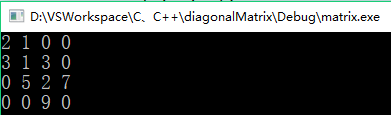五、三角矩阵
下三角矩阵：M是一个下三角矩阵，当且仅当i<j时，M(i,j)=0上三角矩阵：M是一个上三角矩阵，当且仅当i>j时，M(i,j)=0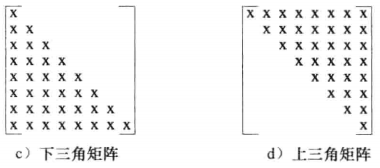编码实现：
在一个n行的下三角矩阵中（见下图），非0区域的第一行有1个元素，第二行有2个元素，......，第n行有n个元素在一个n行的上三角矩阵中（见下图），非0区域的第一行有n个元素，第二行有n-1个元素，......，第n行有1个元素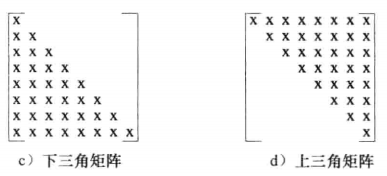总结：下三角矩阵或上三角矩阵中非0区域共有非0元素：n(n+1)/2。一个三角矩阵可以用一个大小为n(n+1)/2的一维数组来表示以下面的下三角矩阵为例：
按行映射：element[0-9]={2,5,1,0,3,1,4,2,7,0}按列映射：element[0-9]={2,5,0,4,1,3,2,1,7,0}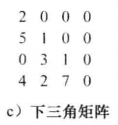下三角矩阵的元素L(i,j)：
如果i<j，则L(i,j)=0如果i>=j，则L(i,j)位于非0区域。如果按行映射，在元素L(i,j)（i>=j）之前分别有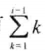个元素位于第1行至第i-1行的非0区域和j-1个元素位于第i行的非0区域拒，共有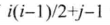个
异常类定义
class illegalParameterValue
{
string message;
public:
illegalParameterValue(const char *theMessage ="Illegal Parameter Value"):message(theMessage) {}
const char *what() {
return message.c_str();
}
};

class matrixIndexOutOfBounds
{
public:
matrixIndexOutOfBounds(string theMessage = "Matrix index out of bounds") :message(theMessage){}
const char *what() {
return message.c_str();
}
private:
string message;
};
类定义
template<typename T>
class lowerTriangularMatrix
{
public:
lowerTriangularMatrix(int theN = 10);
~lowerTriangularMatrix();
void set(int i, int j, T value);
T get(int i, int j)const;
void output(ostream& out) const;
private:
T *element;  //存放矩阵的数组
int n;  //矩阵维度
};
类成员实现
template<typename T>
lowerTriangularMatrix<T>::lowerTriangularMatrix(int theN = 10)
{
if (theN < 1) {
throw illegalParameterValue("Parameter mu be >=1");
}

element = new T[theN*(theN + 1) / 2];
n = theN;
}

template<typename T>
lowerTriangularMatrix<T>::~lowerTriangularMatrix()
{
if (element) {
delete[] element;
element = nullptr;
}
}

template<typename T>
void lowerTriangularMatrix<T>::set(int i, int j, T value)
{
if ((i<1) || (j<1) || (i>n) || (j>n)) {
throw matrixIndexOutOfBounds();
}

if (i >= j) {
element[i*(i - 1) / 2 + j - 1] = value;
}
else {
if (value != 0) {
throw illegalParameterValue("nondiagonal elements must be zero");
}
}
}

template<typename T>
T lowerTriangularMatrix<T>::get(int i, int j)const
{
if ((i<1) || (j<1) || (i>n) || (j>n)) {
throw matrixIndexOutOfBounds();
}

if (i >= j)
return element[i*(i - 1) / 2 + j - 1];
else
return 0;
}

template<typename T>
void lowerTriangularMatrix<T>::output(ostream& out) const
{
for (int i = 1; i <= n; ++i) {
for (int j = 1; j <= n; j++) {
if (i >= j) {
out << element[i*(i - 1) / 2 + j - 1] << " ";
continue;
}
out << "0 ";
}
out << endl;
}
}
演示效果
int main()
{
lowerTriangularMatrix<int> *matrix = new lowerTriangularMatrix<int>(4);
matrix->set(1, 1, 2);
matrix->set(2, 1, 5);
matrix->set(2, 2, 1);
matrix->set(3, 1, 0);
matrix->set(3, 2, 3);
matrix->set(3, 3, 1);
matrix->set(4, 1, 4);
matrix->set(4, 2, 2);
matrix->set(4, 3, 7);
matrix->set(4, 4, 0);

matrix->output(cout);

return 0;
}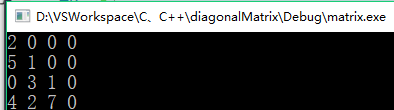六、对称矩阵
对称矩阵：M是一个对称矩阵，当且仅当对于所有的j和j，M(i,j)=M(j,i)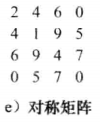编码实现：一个n*n的对称矩阵，可以视为下三角或上三角矩阵，用三角矩阵的表示方法，用一个大小为n(n+1)/2的一维数组来表示。未存储的元素可以用存储的元素来计算
七、总结
代码下载见：https://download.csdn.net/download/qq_41453285/12007285
展开全文特殊矩阵 对三角矩阵 三角矩阵 对称矩阵
• ## 实对称矩阵一定可以对角化

万次阅读 多人点赞 2020-06-28 11:11:44
实对称矩阵一定可以对角化. 最近看共轭梯度下降的时候看到有人的推导里面用到了这个命题. 虽然以前学过, 但是学得很渣, 所以没有自己想过这个命题怎么样成立的. 现在将这些证明过程梳理一下. 实对称矩阵含有n个实根 ...
UTF8gbsn
实对称矩阵一定可以对角化. 最近看共轭梯度下降的时候看到有人的推导里面用到了这个命题. 虽然以前学过, 但是学得很渣, 所以没有自己想过这个命题怎么样成立的. 现在将这些证明过程梳理一下.
实对称矩阵含有n个实根
首先我们来证明一个命题, 实对称矩阵含有n个实根, 注意,n个实根并不一定都是不同的, 可能含有重根. 比如

(

r

−

1

)

2

=

0

(r-1)^2=0

就含有两个重根

r

=

1

r=1

.在计算根数目的时候这个方程的解算两个.
首先, 任意的矩阵

A

\mathbf{A}

,它的特征多项式

∣

A

−

λ

I

∣

=

0

|\mathbf{A}-\lambda\mathbf{I}|=0

是一个

n

n

次多项式(这是很显然的). 由于

n

n

次多项式必定有

n

n

个根(在复数域上). 这个命题暂不证明, 直接使用. 我写过另外一篇文章简要的证明了一下这个定理.  有了上一步的结论, 我们现在只需要证明每一个根

λ

i

\lambda_i

是实根就可以了. 这个证明过程很简单. 假设

λ

i

\lambda_i

是任意根之一, 并且

α

i

\mathbf{\alpha}_i

(当然也是在复数域), 那么根据特征值和特征向量的定义.我们可以得

A

α

i

=

λ

i

α

i

\mathbf{A}\mathbf{\alpha}_i=\lambda_i\mathbf{\alpha}_i

取共轭得

A

α

‾

i

=

λ

‾

i

α

‾

i

\mathbf{A}\mathbf{\overline{\alpha}}_i=\overline{\lambda}_i\mathbf{\overline{\alpha}}_i

再进行转置得, 注意

A

T

=

A

A^T=A

, 对称矩阵.

α

‾

i

T

A

=

λ

‾

i

α

‾

i

T

\mathbf{\overline{\alpha}}_i^T\mathbf{A}=\overline{\lambda}_i\mathbf{\overline{\alpha}}_i^T

右边乘

α

i

\mathbf{\alpha}_i

得

α

‾

i

T

A

α

i

=

λ

‾

i

α

‾

i

T

α

i

\mathbf{\overline{\alpha}}_i^T\mathbf{A}\mathbf{\alpha}_i=\overline{\lambda}_i\mathbf{\overline{\alpha}}_i^T\mathbf{\alpha}_i

再看

A

α

i

=

λ

i

α

i

\mathbf{A}\mathbf{\alpha}_i=\lambda_i\mathbf{\alpha}_i

, 对它左边乘

α

‾

i

T

\overline{\mathbf{\alpha}}_i^{T}

可得

α

‾

i

T

A

α

i

=

λ

i

α

‾

i

T

α

i

\mathbf{\overline{\alpha}}_i^T\mathbf{A}\mathbf{\alpha}_i=\lambda_i\mathbf{\overline{\alpha}}_i^T\mathbf{\alpha}_i

上面两个式子相减得

0

=

(

λ

‾

i

−

λ

i

)

α

‾

i

T

α

i

0=(\mathbf{\overline{\lambda}_i-\lambda_i})\mathbf{\overline{\alpha}}_i^T\mathbf{\alpha}_i

因为,

α

‾

i

T

α

i

\mathbf{\overline{\alpha}}_i^T\mathbf{\alpha}_i

是非0向量.所以我们可得

λ

‾

i

−

λ

i

=

0

\overline{\lambda}_i-\lambda_i=0

. 也就是说

λ

i

\lambda_i

是实数. 又因为

λ

i

\lambda_i

是任意的特征值,所以

A

\mathbf{A}

, 的所有特征值都是实数.
实对称矩阵属于不同特征值的特征向量正交
接下来我们再来证明一个命题,实对称矩阵属于不同特征值的特征向量正交.我们先假设两个不同的特征值位

λ

i

,

λ

j

\lambda_i,\lambda_j

, 他们对应的特征向量为

α

i

,

α

j

\mathbf{\alpha}_i, \mathbf{\alpha}_j

. 假如, 我们定义

(

α

i

,

α

j

)

(\mathbf{\alpha}_i, \mathbf{\alpha}_j)

表示点积. 那么我们可以按照下面的推导.

λ

i

(

α

i

,

α

j

)

=

(

λ

i

α

i

,

α

j

)

=

(

A

α

i

,

α

j

)

=

α

j

T

A

α

i

=

α

i

T

A

α

j

\lambda_i(\mathbf{\alpha}_i, \mathbf{\alpha}_j)=(\lambda_i\mathbf{\alpha}_i, \mathbf{\alpha}_j)=(\mathbf{A\alpha}_i, \mathbf{\alpha}_j)=\mathbf{\alpha_j^TA\alpha_i}=\mathbf{\alpha_i^TA\alpha_j}

λ

j

(

α

i

,

α

j

)

=

(

α

i

,

λ

j

α

j

)

=

(

α

i

,

A

α

j

)

=

α

i

T

A

α

j

\lambda_j(\mathbf{\alpha}_i, \mathbf{\alpha}_j)=(\mathbf{\alpha}_i, \lambda_j\mathbf{\alpha}_j)=(\mathbf{\alpha}_i, \mathbf{A\alpha}_j)=\mathbf{\alpha_i^TA\alpha_j}

下相减得

(

λ

i

−

λ

j

)

(

α

i

,

α

j

)

=

0

(\lambda_i-\lambda_j)(\mathbf{\alpha}_i, \mathbf{\alpha}_j)=0

, 又因为

λ

i

≠

λ

j

⇒

α

i

T

⋅

α

j

=

0

\lambda_i\neq \lambda_j \Rightarrow \mathbf{\alpha}_i^T\cdot \mathbf{\alpha}_j=0

, 也就是正交成立.
实对称矩阵可对角化
这里我们使用归纳法来证明.
首先假设

n

=

1

n=1

.

A

=

a

11

\mathbf{A}=a_{11}

. 这个不证自明.  假设

n

=

k

−

1

n=k-1

, 命题撤成立.  现在

n

=

k

n=k

, 我们假设其中一个特征值位

λ

1

\lambda_1

, 那么我可以利用第一个特征值对应的特征向量构造一组

R

n

R^n

的正交基.

T

=

(

η

1

,

η

2

,

⋯

,

η

n

)

T=(\eta_1, \eta_2,\cdots, \eta_n)

. 那么我们可以得

T

−

1

A

T

=

(

T

−

1

λ

1

η

1

,

T

−

1

A

η

2

,

⋯

,

T

−

1

A

η

n

)

T^{-1}AT=(T^{-1}\lambda_1\eta_1, T^{-1}A\eta_2, \cdots, T^{-1}A\eta_n)

又因为

T

−

1

T

=

I

T^{-1}T=I

, 那么, 我们可以得

T

−

1

η

1

=

ε

1

T^{-1}\eta_1=\mathbf{\varepsilon}_1

. 那么可得

T

−

1

A

T

=

(

λ

1

α

0

B

)

T^{-1}AT=\left( \begin{array}{cc} \lambda_1 & \mathbf{\alpha} \\ \mathbf{0} & \mathbf{B} \end{array} \right)

由于

A

A

, 是一个实对称矩阵, 那么

T

−

1

A

T

T^{-1}AT

也是一个实对称矩阵.进而

α

=

0

\mathbf{\alpha}=\mathbf{0}

. 由此可见

B

B

也是一个

(

k

−

1

)

×

(

k

−

1

)

(k-1)\times (k-1)

的实对称矩阵. 按照假设, 它是可以对角化的.现在假设.

T

2

−

1

B

T

2

=

d

i

a

g

{

λ

2

,

λ

3

,

⋯

,

λ

n

}

T_2^{-1}BT_{2}=diag\{\lambda_2, \lambda_3, \cdots, \lambda_n\}

并设

T

f

=

T

(

1

0

0

T

2

)

T_f=T\left( \begin{array}{cc} 1 & 0 \\ 0 & T_2 \end{array} \right)

那么

T

f

−

1

A

T

f

=

(

1

0

0

T

2

)

−

1

T

−

1

A

T

(

1

0

0

T

2

)

=

(

1

0

0

T

2

−

1

)

(

λ

1

0

0

B

)

(

1

0

0

T

2

)

T^{-1}_fAT_{f}=\left( \begin{array}{cc} 1 & 0 \\ 0 & T_2 \end{array} \right)^{-1}T^{-1}AT\left( \begin{array}{cc} 1 & 0 \\ 0 & T_2 \end{array} \right)=\left( \begin{array}{cc} 1 & 0 \\ 0 & T_2^{-1} \end{array} \right)\left( \begin{array}{cc} \lambda_1 & 0 \\ 0 & B \end{array} \right)\left( \begin{array}{cc} 1 & 0 \\ 0 & T_2 \end{array} \right)

=

(

λ

1

0

0

T

2

−

1

B

T

2

)

=

d

i

a

g

{

λ

1

,

λ

2

,

⋯

,

λ

n

}

=\left( \begin{array}{cc} \lambda_1& 0 \\ 0 & T_2^{-1}BT_{2} \end{array} \right)=diag\{\lambda_1,\lambda_2, \cdots, \lambda_n\}

至此, 原式得证. 也就是说实对称矩阵一定可以对角化. 反过来也说明实对称矩阵的特征多项式的代叔重述等于几何重数.
展开全文• ，编写程序实现计算对角线元素之和。 我给大家写在纸上，大家来一起观察。 你会发现，奇数型矩阵（a）的对角线上的数全是奇数，(你还可以拿张纸自行试一下a、a、a…)那么我们可以利用奇数...
• pytorch 取对角线元素/矩阵对角线元素置0 使用 torch.diag 取对角线元素，使用 torch.diag_embed() 恢复维度 import torch a = torch.randn(3, 3) print(a) tensor([[ 0.7594, 0.8073, -0.1344], [-1.7335, -0....
• ## 线性代数笔记8：矩阵的对角化

万次阅读 多人点赞 2018-04-02 21:33:36
本文主要讲矩阵对角化的证明及应用。 矩阵对角化条件 定义一：若存在可逆矩阵SSS，使得S−1ASS−1ASS^{-1}AS为对角矩阵，则称为矩阵AAA是可对角化的（diagonalized）。 设n×nn×nn\times n矩阵有nnn个线性...特征值 矩阵 线性代数
• 原来，实对称矩阵对角化是为解决解析几何中二次曲面是何类型而提出的，因为二次曲面的方程可以写成(x,y,z)A(xyz)(x,y,z)A\begin{pmatrix}x\\y\\z\end{pmatrix}(x,y,z)A⎝⎛​xyz​⎠⎞​的形式，而这里的A就是一个实...
• Python对二维矩阵沿主对角线（次对角线）翻转变换代码实现 目录Python对二维矩阵沿主对角线（次对角线）翻转变换代码实现1. 原始数据以及图示2. 主对角线翻转及图示3. 次对角线翻转结果4. 完整代码，如有疑问，欢迎...python 数组 矩阵
• python 对角线矩阵Some problems in linear algebra are mainly concerned with diagonal elements of the matrix. For this purpose, we have a predefined function numpy.diag(a) in NumPy library package which...python numpy 机器学习 线性代数 人工智能
• np.diagonal(a, offset=0, axis1=0, axis2=1) ...当offset=1时，对角线向上移动1个位置，offset=2，移动两个位置，以此类推。当offset取负值时，向下移动相应的位置数量。见下： arr =np.arange(9...python numpy 数据结构
• R语言产生各种类型的矩阵及矩阵运算R语言产生一般的矩阵R语言产生单位阵R语言产生次对角阵R语言矩阵的常见运算 R语言产生一般的矩阵 # 依行排列，产生3行5列的矩阵 A = matrix(c(1:15),3,5,byrow=T) R语言产生单位...线性代数 矩阵 数据挖掘
• ## 矩阵可对角化条件

千次阅读 2019-08-31 16:21:28
除了对角矩阵元素的排列次序可变动， A A A 的相似标准形是唯一的 定理2 设 λ 1 , λ 2 \lambda_1,\lambda_2 λ 1 ​ , λ 2 ​ 是数域 K K K 上的 n n n 级矩阵 A A A 得到不同特征值， α 1 , α 2 , . . ....
• ***知识点：理解对角线与熟悉二维数组的下标。 二维数组本质上是以数组作为数组元素的数组，即“数组的数组”，类型说明符 数组名[常量表达式][常量表达式]。二维数组又称为矩阵，行列数相等的矩阵称为方阵。 ...c++
• 这次来实现三对角线方程组的追赶法，追赶法的本质还是高斯消元法，而且是没选主元的高斯消元法，只是因为Ax=b中系数矩阵A非常特殊，所以就可以采用相对特殊的方法来解方程组。同样，按照常规的步骤，先分析什么是...
• 行严格对角占优矩阵——一道矩阵代数作业题 今年（2018年）11月20日的矩阵代数课上老师布置了一道课后作业题，题目如下： 已知矩阵 A=(aij)∈Cn×nA=\left(a_{ij}\right)\in \mathbb{C}^{n\times n}A=(aij​)∈Cn×n...
• 本文从矩阵为什么要对角化讲到为什么不能对角化，解释不能对角化是什么意思可参看视频，主要思想表述如下： 矩阵相似对角化与不能对角化的解释 矩阵相似对角化的进一步理解，几何加本质 对一般矩阵的研究转化...机器学习 人工智能 计算机视觉 深度学习 matlab
• ## C语言-矩阵对角线

千次阅读 2021-03-08 22:46:08
C语言的矩阵对角线 问： 求矩阵对角线的和？ 所谓矩阵就是一个多维数组比较好理解的称呼，是线性存储的。 求矩阵对角线就要知道它的特点， 首先正对角线也就是这么 \ 一条线，它的特点是i=j; 反对角线 / 的特点是 i ...c语言
• 我们在控制移动机器人时，比较核心和...双舵轮的AGV能有效的弥补上述问题，这种双舵轮的AGV一般使用对角安装的方式安装，具有很高的灵活性，不仅承载能力强，而且可以全向移动，是一种非常不错的重载AGV机构方案，在...
• 给定一个含有 M x N 个元素的矩阵（M 行，N 列），请以对角线遍历的顺序返回这个矩阵中的所有元素，对角线遍历如下图所示。 示例: 输入: [ [ 1, 2, 3 ], [ 4, 5, 6 ], [ 7, 8, 9 ] ] 输出: [1,2,4,7,5,3,6,8,9]...数组 数据结构与算法
• int[][] Sort(int[][] input) 测试用例： 输入：      ...注意输出的数字是沿对角排序, 注意处理2维数组的下标. 输入可以是hard code的常量,如果是这样,请将输入也打印到控制台.java
• ## 对角矩阵

千次阅读 2019-05-15 22:53:27
设是n维线性空间V的一个线性变换，的矩阵可以在某一组基下为对角矩阵的充分必要条件是，有n个线性无关的特征向量。 定理2 属于不同特征值的特征向量是线性无关的。 推论1 如果在n维线性空间V中，线性变换的特征...
• ​∣u n​∣​⎠⎞​ 相似对角化 条件：A有n个线性无关的特征向量，即：P (特征向量矩阵) 可逆 A含有n个不同的特征值，或者特征值中的k重特征值对应有k个线性无关的特征向量，则A可以相似对角化 实现矩阵对角化 main...
• 线性代数 矩阵相似对角化的理解 矩阵的相似对角化，是一种基变换，或者说是坐标系变换，本质上是将线性变换在原坐标系（标准坐标系）中的表示变换为在新的坐标系下的表示，而这个新的坐标系刚好是由线性变换的一组...
• 最近在网上看到这样一道面试题：二维数组（N*N），沿对角线方向，从右上角打印到左下角如N=4： 4*4二维数组 { 1 2 3 4 } { 5 6 7 8 } { 9 10 11 12 } {13 14 15 16 } 打印顺序 4 3 8 2 7 12 1 6 11 16 5...二维数组 数组算法 java
• 实现的最终结果如下：...对角线的实现 linear-gradient(45deg,red 30px,pink 0); （2）-135deg -45deg 45deg 135deg 也就是说，四个角斜切的方位是按照不同的度数来完成的。 代码如下： <...
• //二维数组主对角线与次对角线输出 #include <stdio.h> #include <stdlib.h> #define N int main() { int arr; #if 0 //int arr //省3等价于省了一维数组的大小 //省4等价于省了类型的...c语言
• 一、生成对角矩阵的基本用法 1、diag(a) 使用diag(a)命令生成对角矩阵，a为某个向量，如下所示： 2、diag(a,i) 使用diag(a,i)命令生成，a为某个向量，i为a向量相对主对角线偏移的列数（向上为正，向下为负）。...MATLAB
• 对角线矩阵的伴随矩阵Here is the C program to find sum of diagonal of a square matrix. 这是C语言程序，用于查找方矩阵的对角线之和。 #include<stdio.h> int main() { int i,j,n,d1=0,d2=0,a; ...c语言 算法 python 小程序 c++...